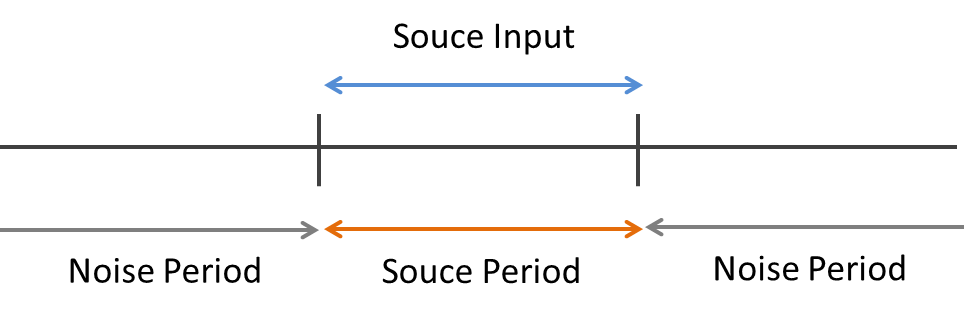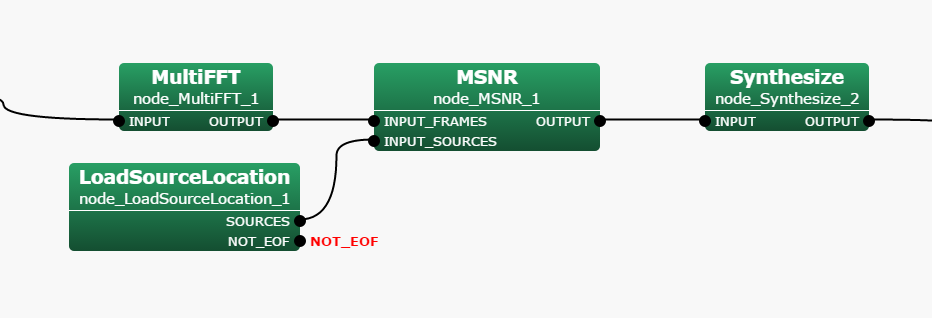6.3.9 MSNR

6.3.9.1 Outline of the node

Perform sound source separation using the method of maximum SNR (Maximum Signal-to-Noise Ratio). In this algorithm, perform sound source separation by updating the separation matrix so that the ratio of the gain in the target sound source direction and the gain in the known noise direction is maximized. Transfer function information from the sound source to the microphones in advance is not required; however, the section information on the sound source (detection result of the utterance section) is necessary.

Node inputs are:

• Multi-channel complex spectrum of mixed sound,

• Direction of localized sound sources.

Note outputs are a set of complex spectrum of each separated sound.

6.3.9.2 Necessary files

No files are required.

6.3.9.3 Usage

When to use

This node is used to perform sound source separation on the sound source direction originated using a microphone array. The sound source direction can be either a value estimated by sound source localization or a constant value. Since this node uses the ratio of the gain in the target sound source and the gain in the known noise, it requires the speech period information of the known noise. This node treats the time period during which no sound source direction data is input as the period with noise, the Noise Period.Figure 6.72: Noise Period vs Source Period

Typical connection

Figure 6.73 shows a connection example of the MSNR . The node has two inputs as follows:

1. INPUT_FRAMES takes a multi-channel complex spectrum containing the mixture of sounds produced by for example MultiFFT ,

2. INPUT_SOURCES takes the results of sound source localization produced by for example LocalizeMUSIC or ConstantLocalization ,

The output is the separated signals.Figure 6.73: Example of connection of the MSNR

6.3.9.4 Input-output and property of the node

Input

INPUT_FRAMES

: Matrix<complex<float> >  type. Multi-channel complex spectra. Corresponding to the complex spectrum of input waveform from each microphone, the rows correspond to the channels and the columns correspond to the frequency bins.

INPUT_SOURCES

: Vector<ObjectRef>  type. A Vector array of the Source type object in which sound source localization results are stored. Typically, takes the output of SourceIntervalExtender connected to SourceTracker .

Output

OUTPUT

: Map<int, ObjectRef>  type. A pair containing the sound source ID and the complex spectrum of the separated sound (Vector<complex<float> > type). Output as many as the number of sound sources .

Parameter

LENGTH

: int  type. Analysis frame length [samples], which must be equal to the values at a preceding node (e.g. AudioStreamFromMic or the MultiFFT ). The default is 512.

: int  type. Shift length of a frame [samples], which must be equal to the values at a preceding node (e.g. AudioStreamFromMic or the MultiFFT ). The default is 160.

SAMPLING_RATE

: int  type. Sampling frequency of the input waveform [Hz]. The default is 16000.

LOWER_BOUND_FREQUENCY

: int  type. This parameter is the minimum frequency used when separation processing is performed. Processing is not performed for frequencies below this value and the value of the output spectrum is zero then. The user designates a value in the range from 0 to half of the sampling frequency.

UPPER_BOUND_FREQUENCY

: int  type. This parameter is the maximum frequency used when separation processing is performed. Processing is not performed for frequencies above this value and the value of the output spectrum is zero then. LOWER_BOUND_FREQUENCY $<$ UPPER_BOUND_FREQUENCY must be maintained.

DECOMPOSITION_ALGORITHM

: string  type. The decomposition algorithm to perform sound source separation. GEVD represents generalized eigenvalue decomposition. GSVD represents generalized singular value decomposition. GEVD has better noise suppression performance than GSVD whereas GEVD costs longer calculation time than GSVD. Select the appropriate algorithm according to the purpose and the computer environment.

ALPHA

: float  type. The stepsize for updating correlation matrices. The default value is 0.99.

ENABLE_DEBUG

: bool  type. The default value is false. Setting the value to true outputs the separation status to the standard output.

Table 6.58: Parameter list of MSNR
 Parameter list Type Default value Unit Description LENGTH 512 [pt] Analysis frame length. ADVANCE 160 [pt] Shift length of frame. SAMPLING_RATE 16000 [Hz] Sampling frequency. LOWER_BOUND_FREQUENCY 0 [Hz] The minimum frequency value used for separation processing. UPPER_BOUND_FREQUENCY 8000 [Hz] The maximum frequency value used for separation processing. DECOMPOSITION_ALGORITHM GEVD The decomposition algorithm. ALPHA 0.99 The stepsize for updating correlation matrices. ENABLE_DEBUG false Enable or disable to output the separation status to standard output.

6.3.9.5 Details of the node

Technical details: Please refer to the following reference for the details.

Brief explanation of sound source separation: Table 6.48 shows the notation of variables used in sound source separation problems. Since the source separation is performed frame-by-frame in the frequency domain, all the variable is computed in a complex field. Also, the separation is performed for all $K$ frequency bins ($1 \leq k \leq K$). Here, we omit $k$ from the notation. Let $N$, $M$, and $f$ denote the number of sound sources and the number of microphones, and the frame index, respectively.

Table 6.59: Notation of variables
 Variables Description $\boldsymbol {S}(f) = \left[S_1(f), \dots , S_ N(f)\right]^ T$ Complex spectrum of target sound sources at the $f$-th frame. $\boldsymbol {X}(f) = \left[X_1(f), \dots , X_ M(f)\right]^ T$ Complex spectrum of a microphone observation at the $f$-th frame, which corresponds to INPUT_FRAMES. $\boldsymbol {N}(f) = \left[N_1(f), \dots , N_ M(f)\right]^ T$ Complex spectrum of added noise. $\boldsymbol {H} = \left[ \boldsymbol {H}_1, \dots , \boldsymbol {H}_ N \right] \in \mathbb {C}^{M \times N}$ Transfer function matrix from the $n$-th sound source ($1 \leq n \leq N$) to the $m$-th microphone ($1 \leq m \leq M$) $\boldsymbol {K}(f) \in \mathbb {C}^{M \times M}$ Correlation matrix of known noise. $\boldsymbol {W}(f) = \left[ \boldsymbol {W}_1, \dots , \boldsymbol {W}_ M \right] \in \mathbb {C}^{N \times M}$ Separation matrix at the $f$-th frame. $\boldsymbol {Y}(f) = \left[Y_1(f), \dots , Y_ N(f)\right]^ T$ Complex spectrum of separated signals.

Use the following linear model for the signal processing:

 $\displaystyle \boldsymbol {X}(f)$ $\displaystyle =$ $\displaystyle \boldsymbol {H}\boldsymbol {S}(f) + \boldsymbol {N}(f) \label{eq:beamforming-observation}$ (82)

The purpose of the separation is to estimate $\boldsymbol {W}(f)$ based on the following equation:

 $\displaystyle \boldsymbol {Y}(f)$ $\displaystyle =$ $\displaystyle \boldsymbol {W}(f)\boldsymbol {X}(f) \label{eq:Beamforming-separation}$ (83)

so that $\boldsymbol {Y}(f)$ is getting close to $\boldsymbol {S}(f)$.

Assuming that the correlation matrix of the target sound signal is $\boldsymbol {R}_{ss}(f)$ and the correlation matrix of the noise signal is $\boldsymbol {R}_{nn}(f)$, the evaluation function $J_{\textrm{MSNR}}(\boldsymbol {W}(f))$ for updating the separation matrix is expressed as follows.

 $\displaystyle J_{\textrm{MSNR}}(\boldsymbol {W}(f))$ $\displaystyle =$ $\displaystyle \frac{\boldsymbol {W}(f))\boldsymbol {R}_{ss}(f)\boldsymbol {W}(f))^ H}{\boldsymbol {W}(f))\boldsymbol {R}_{nn}(f)\boldsymbol {W}(f))^ H} \label{eq:MSNR}$ (84)

In the MSNR , obtain $\boldsymbol {W}(f)$ that maximizes $J_{\textrm{MSNR}}(\boldsymbol {W}(f))$ using generalized eigenvalue decomposition or generalized singular value decomposition.

Here, the correlation matrix $\boldsymbol {R}_{ss}(f)$ of the target sound signal is updated as follows using the correlation matrix $\boldsymbol {R}_{xx}(f)$ obtained from the signal in the period during which the INPUT_SOURCES input terminal receives target sound source direction data.

 $\displaystyle \boldsymbol {R}_{ss}(f+1)$ $\displaystyle =$ $\displaystyle \alpha \boldsymbol {R}_{ss}(f) + (1-\alpha )\boldsymbol {R}_{xx}(f) \label{eq:MSNR-Rss}$ (85)

On the other hand, the correlation matrix $\boldsymbol {R}_{nn}(f)$ of the noise signal is updated as follows using the correlation matrix $\boldsymbol {R}_{xx}(f)$ obtained from the signal in the period (the Noise Period) during which the INPUT_SOURCES input terminal receives no data.

 $\displaystyle \boldsymbol {R}_{nn}(f+1)$ $\displaystyle =$ $\displaystyle \alpha \boldsymbol {R}_{nn}(f) + (1-\alpha )\boldsymbol {R}_{xx}(f) \label{eq:MSNR-Rnn}$ (86)

The $\alpha$ in the equation (85) and the equation (86) can be specified in the ALPHA property.

$\boldsymbol {W}(f)$ is updated by $\boldsymbol {R}_{ss}(f)$ and $\boldsymbol {R}_{nn}(f)$ and so separated.

Trouble shooting: Basically, same as GHDSS node troubleshooting.

6.3.9.6 Reference

• P. W. Howells, ’Intermediate Frequency Sidelobe Canceller’, U.S. Patent No.3202990, 1965.